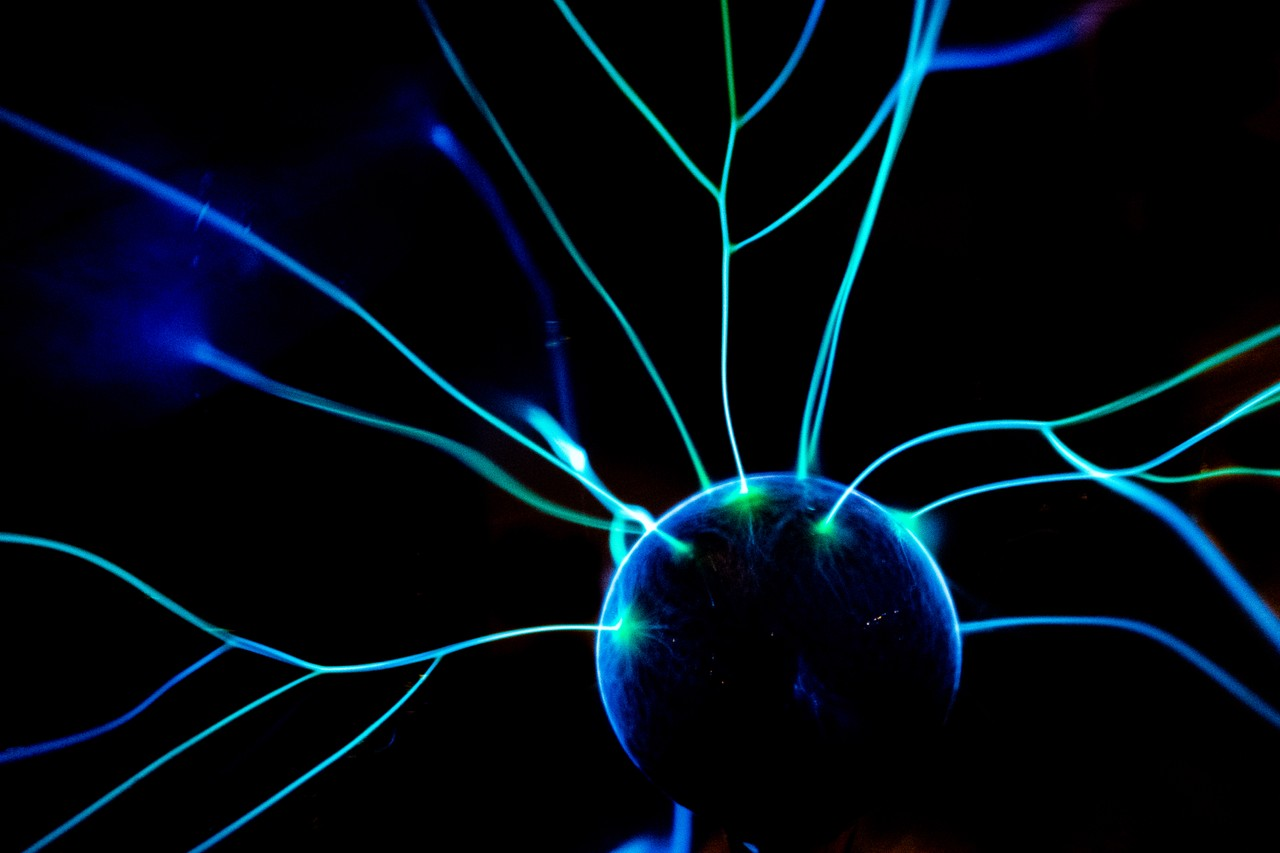AI and Deep Learning for Java (DL4J) provides an extensive suite of tools for building production-grade deep-learning applications. Scalability and performance can be achieved using distributing training, re-enforcement learning and inference across CPU and GPU clusters. Integration with Apache Kafka for data streaming, Apache Spark for distributed computing and Hadoop for large-scale data analytics makes DL4J suited for enterprise-level AI applications.

This course provides a solid foundation in the concepts of neural-network based Artificial Intelligence (AI) and deep learning and in developing scalable deep learning solutions using the DL4J framework.

The course is for software developers, software engineers, data engineers and scientists who would like to augment their Java-based systems with deep learning features.

• Java programming
• Basic mathematics

## Course content

• Neural Network Basics
• Artificial Neurons, Activation functions and the Perceptron
• Neural network structures and their uses
• Multi-layer perceptrons (MLPs)
• Convolutional neural networks (CNNs)
• Recurrent neural networks (RNNs)
• LSTM networks
• Training/Learning Algorithms
• Training = global optimization
• Backpropagation
• Genetic algorithms, Simulated anealing, swarm optimization, …
• Reinforcement learning
• Understanding and using ND4J and DL4J
• Overview of ND4J and DL4J
• Creating a simple DL4J application
• Creating and training a perceptron with ND4J
• Single-hidden layer perceptrons
• Multi-Layer Perceptrons (MLPs) and Deep-Learning in DL4J
• The need for deep-learning
• Constructing a MLP in DL4J
• Data preparation and normalization
• Training a deep-learning neural network
• Using the training MLP for inference
• Optimizing the network for the problem at hand
• An MLP example application
• Convolutional Neural Networks (CNNs) in DL4J
• Limitations of feed-forward networks
• Translation, rotation and scaling invariance
• Overfitting
• Convolutional neural networks and transformation of the input signals
• The structure of a convolutional neural network
• Dimensionality reduction through feature extraction
• The classifier part of a CNN
• Constructing a CNN in DL4J
• Training CNNs
• Using the CNN for inference
• An example CNN application
• Basic Recurrent Neural Networks (RNN)
• The structure of an RNN
• Time series prediction and language processing using RNNs
• Modifying backpropagation for RNNs
• Causes of exploding and vanishing gradient problems
• Solving the exploding gradient problem
• Long-Short-Term Memory (LSTM) networks in DL4J
• Solving the vanishing gradient problem with an LSTM architecture
• Constructing an LSTM in DL4J
• Using LSTMs to make predictions
• Optimizing LSTMs
• Using LSTM for stock market predictions
• Gated Recurrent Units (GRUs) in DL4J
• Reducing the number of training parameters through GRUs
• Benefits and disadvantages of GRUs
• Constructing GRUs in DL4J
• Distributed training
• Using Apache Spark computing clusters
• Using GPU grids

## Certification

The Domain-Oriented Analysis and Design course using UML & URDAD is part of the

Scroll to Top